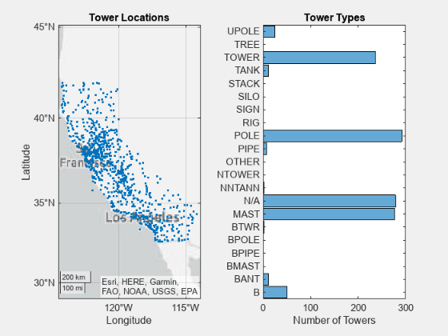## Develop Charts With Polar Axes, Geographic Axes, or Multiple Axes

For charts you develop as a subclass of the `ChartContainer` base class, the `getAxes` method provides a way to support a single Cartesian axes object. If you want to support polar axes, geographic axes, or multiple axes, you must create and configure the axes as children of a `TiledChartLayout` object, which is stored in the chart object.

### Create a Single Polar or Geographic Axes Object

To include a single polar axes or geographic axes object in your chart:

1. Define a private property to store the axes.

2. In the `setup` method:

For example, here is a basic class that contains a polar axes object.

```classdef SimplePolar < matlab.graphics.chartcontainer.ChartContainer properties(Access = private,Transient,NonCopyable) PolarAx matlab.graphics.axis.PolarAxes end methods(Access = protected) function setup(obj) % Get the layout and create the axes tcl = getLayout(obj); obj.PolarAx = polaraxes(tcl); % Other setup code % ... end function update(obj) % Update the chart % ... end end end```

### Create a Tiling of Multiple Axes Objects

To display a tiling of multiple axes:

1. Define private properties that store the axes objects. You can also define one property that stores an array of axes objects.

2. In the `setup` method:

• Call the `getLayout` method to get the `TiledChartLayout` object.

• Set the `GridSize` property of the `TiledChartLayout` object so that it has at least one tile for each of the axes.

• Call the `axes`, `polaraxes`, or `geoaxes` function to create the axes objects, and specify the `TiledChartLayout` object as the parent object.

• Move each of the axes to the desired tile by setting the `Layout` property on each axes object. By default, the axes appear in the first tile.

For example, here is a basic class that contains two Cartesian axes:

```classdef TwoAxesChart < matlab.graphics.chartcontainer.ChartContainer properties(Access = private,Transient,NonCopyable) Ax1 matlab.graphics.axis.Axes Ax2 matlab.graphics.axis.Axes end methods(Access = protected) function setup(obj) % Get the layout and set the grid size tcl = getLayout(obj); tcl.GridSize = [2 1]; % Create the axes obj.Ax1 = axes(tcl); obj.Ax2 = axes(tcl); % Move the second axes to the second tile obj.Ax2.Layout.Tile = 2; end function update(obj) % Update the chart % ... end end end```

### Example: Chart Containing Geographic and Cartesian Axes

This example shows how to define a class of charts for visualizing geographic and categorical data using two axes. The left axes contains a map showing the locations of several cellular towers. The right axes shows the distribution of the towers by category.

The following `TowerChart` class definition shows how to:

• Define a public property called `TowerData` that stores a table.

• Validate the contents of the table using a local function called `mustHaveRequiredVariables`.

• Define two private properties called `MapAxes` and `HistogramAxes` that store the axes.

• Implement a `setup` method that gets the `TiledChartLayout` object, specifies the grid size of the layout, and positions the axes.

To define the class, copy this code into the editor and save it with the name `TowerChart.m` in a writable folder.

```classdef TowerChart < matlab.graphics.chartcontainer.ChartContainer properties TowerData (:,:) table {mustHaveRequiredVariables} = table([],... [],[],'VariableNames',{'STRUCTYPE','Latitude','Longitude'}) end properties (Access = private,Transient,NonCopyable) MapAxes matlab.graphics.axis.GeographicAxes HistogramAxes matlab.graphics.axis.Axes ScatterObject matlab.graphics.chart.primitive.Scatter HistogramObject matlab.graphics.chart.primitive.categorical.Histogram end methods (Access = protected) function setup(obj) % Configure layout and create axes tcl = getLayout(obj); tcl.GridSize = [1 2]; obj.MapAxes = geoaxes(tcl); obj.HistogramAxes = axes(tcl); % Move histogram axes to second tile obj.HistogramAxes.Layout.Tile = 2; % Create Scatter and Histogram objects obj.ScatterObject = geoscatter(obj.MapAxes,NaN,NaN,'.'); obj.HistogramObject = histogram(obj.HistogramAxes,categorical.empty,... 'Orientation','horizontal'); % Add titles to the axes title(obj.MapAxes,"Tower Locations") title(obj.HistogramAxes,"Tower Types") xlabel(obj.HistogramAxes,"Number of Towers") end function update(obj) % Update Scatter object obj.ScatterObject.LatitudeData = obj.TowerData.Latitude; obj.ScatterObject.LongitudeData = obj.TowerData.Longitude; % Get tower types from STRUCTYPE table variable towertypes = obj.TowerData.STRUCTYPE; % Check for empty towertypes before updating histogram if ~isempty(towertypes) obj.HistogramObject.Data = towertypes; obj.HistogramObject.Categories = categories(towertypes); else obj.HistogramObject.Data = categorical.empty; end end end end function mustHaveRequiredVariables(tbl) % Return error if table does not have required variables assert(all(ismember({'STRUCTYPE','Latitude','Longitude'},... tbl.Properties.VariableNames)),... 'MATLAB:TowerChart:InvalidTable',... 'Table must have STRUCTYPE, Latitude, and Longitude variables.'); end ```

After saving the class file, load the table stored in `cellularTowers.mat`. Then create an instance of the chart by passing the table to the `TowerChart` constructor method as a name-value pair argument.

```load cellularTowers.mat TowerChart('TowerData',cellularTowers); ```